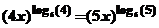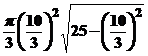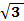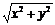WORCESTER POLYTECHNIC INSTITUTE

TWENTY-FIRST ANNUAL INVITATIONAL MATH MEET

OCTOBER 21, 2008

TEAM EXAM QUESTION SHEET WITH ANSWERS

1.       Suppose  a  and  b  are digits (integers from 0 through 9).  What number divides  abba  for all choices of  a  and  b?

Ans:  11

2.      Find all points  (x,y)  in the Euclidean plane satisfying  F(x,y) = 0  where

F(x,y) = xy4 – y4 + x3 y2 – x2 y2 – x3 + x2 – x +1

Ans:  union of the lines:  x = 1, y = 1  and  y= -1.

3.      Find  x > 0  satisfying the following equationAns:  1/20

4.      Arithmetic series sum:  200 + 205 + 210 + 215 + . . . 2745

Ans:  750975

5.      Factor as completely as possible over the reals the following polynomial:

x5 + x4 + 4 x3 – x2 – 19 x + 14

Ans:  (x-1)2(x+2)(x2 + x + 7)

6.      A right circular cone is formed by cutting up a circular piece of paper which is 10 units in diameter.  A sector of angular measure  2Π/3  radians is removed and the remaining paper formed into the cone.  What is its volume?

Ans:7.      Hoodsie the cow is tethered to a corner of a barn which is 20′ by 40′.  Her rope is 60′ long.  How much grazing area does she have?  No barn doors are open.

Ans:  3200Pi

8.      How many zeroes are at the end of the expansion of  31!

Ans:  7

9.      A circle inscribed in an equilateral triangle and a square inscribed in the circle.  What is the ratio of the area of the triangle to that of the square?

Ans:  3:2

10.   What is the minimum value ofif  5x + 12y = 60?

Ans:  60/13

11.   The sum of an infinite geometric series with  -1< r <  1  as its common ratio, is 15.  The sum of the squares of the terms in this series is 45.  What is the first term in this series?

Ans:   a = 5

12.   How many permutations of the letters  D,O,R,E,M,I  do not contain the word DO, RE, MI; that is none of the words DO, RE and MI appears as consecutive letters?

Ans:  426

13.   For some real number  r,  the polynomial  8x3 +4x2 - 42x - 45  is divisible by  (x – r)2.  What is  r ?

Ans:  3/2

14.   How many real solutions are there to the equation:  x256 – 25632 = 0 ?

Ans:  2